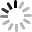# SVG D3.js - 序數比例尺 ( ordinal )• ordinal.domain
• ordinal.range
• ordinal.rangePoints
• ordinal.rangeRoundPoints
• ordinal.rangeBands
• ordinal.rangeRoundBands
• ordinal.rangeBand
• ordinal.rangeExtent
• ordinal.copy

``````var data=[
{x:'a',y:135},
{x:'b',y:138},
{x:'c',y:132},
{x:'d',y:121},
{x:'e',y:126},
{x:'f',y:140},
{x:'g',y:123},
{x:'h',y:89},
{x:'i',y:79},
{x:'j',y:83}
];
``````

``````var s = d3.select('svg');

s.attr({
'width': width,
'height': 200,
}).style({
'border':'1px solid #000'
});
``````

## ordinal.domain([values])

ordinal 的 domain 內所帶入的值，與 linear 有所不同，linear 帶入的是一個數字範圍，而 ordinal.domain 內所帶入的是一串陣列值，陣列的第一個值會對應到第一個元素的的輸出範圍，第二個則會對應到第二個範圍，依此類推。

## ordinal.range([values])

``````var height = 120,
width = 200;

var scaleX1 = d3.scale
.ordinal()
.domain(data.map(function(d){return(d.x);}))
.range([0, width]);

var scaleX2 = d3.scale
.ordinal()
.domain(data.map(function(d){return(d.x);}))
.range([0,20,40,60,80,100,120,140,160,180]);

var scaleY = d3.scale
.linear()
.domain([0,150])
.range([height, 0]);

var line1 = d3.svg.line()
.x(function(d) {
return scaleX1(d.x);
}).y(function(d) {
return scaleY(d.y);
});

var line2 = d3.svg.line()
.x(function(d) {
return scaleX2(d.x);
}).y(function(d) {
return scaleY(d.y);
});

s.append('path')
.attr({
'd':line1(data),
'stroke':'#09c',
'fill':'none'
});

s.append('path')
.attr({
'd':line2(data),
'stroke':'#f66',
'fill':'none',
'transform': 'translate(0,80)'
});
````````````var scaleX = d3.scale
.ordinal()
.domain(data.map(function(d){return(d.x);}))
.rangePoints([0, width]);
````````````var scaleX = d3.scale
.ordinal()
.domain(data.map(function(d){return(d.x);}))
.rangePoints([0, width],1);
````````````var scaleX1 = d3.scale
.ordinal()
.domain(data.map(function(d){return(d.x);}))
.rangePoints([0, width]);

var scaleX2 = d3.scale
.ordinal()
.domain(data.map(function(d){return(d.x);}))
.rangeRoundPoints([0, width]);
``````padding 的值，根據 d3.js 的定義是 0 到 1 之間的數字，而 padding 的實際大小是該 step 點經過 range 之後的數值乘以這個 padding 值，恩，沒錯，講得非常模糊，舉例來說，是我把十個點，放在寬度 100px 的範圍內，把 padding 設為 1，會出現 11.111111 的數值，因為 rangeBands 自的真諦是讓每段 rangeBand 長度相等，而且之間有相等的空隙，當我們把 padding 設為 1，換個角度思考，其實就是利用 padding 補滿一段 bands 的長度，因此 padding 的值就是 10/9=1.11111111。( 範例：svg-d3-08-ordinal-demo6.html )``````var scaleX = d3.scale
.ordinal()
.domain(data.map(function(d){return(d.x);}))
.rangeBands([0, width],4,0);
````````````var scaleX = d3.scale
.ordinal()
.domain(data.map(function(d){return(d.x);}))
.rangeBands([0, width],1);
````````````var scaleX = d3.scale
.ordinal()
.domain(data.map(function(d){return(d.x);}))
.rangeRoundBands([0, width],4,0);
``````## ordinal.rangeBand()

``````console.log(scaleX.rangeBand());
``````

## ordinal.rangeExtent()

``````console.log(scaleX.rangeExtent());
``````

## 小結